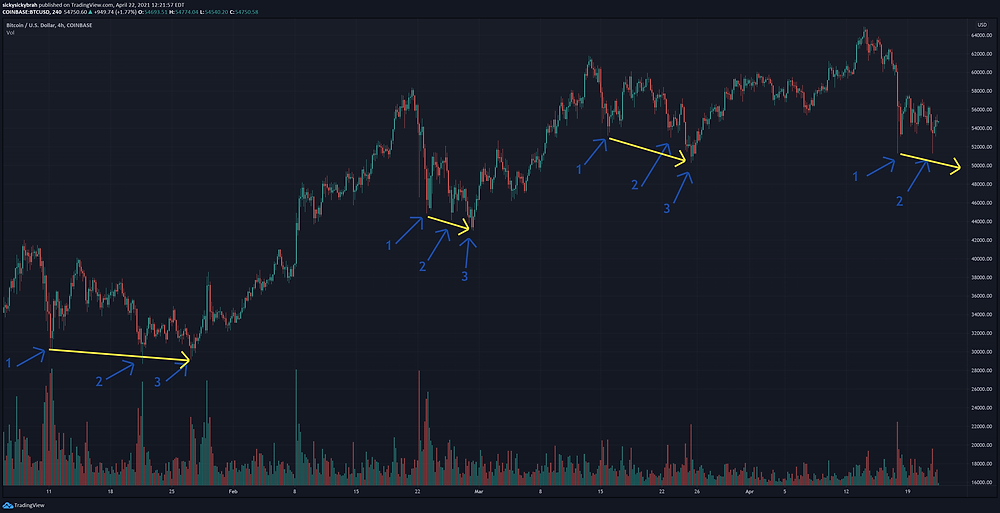Search
•Sir Baller

# April 2021 Updated Bitcoin Price Analysis

*Special thanks to Akin Grace for discussions and ideas regarding Bitcoin trend analysis!

In my last post we analyzed Bitcoin's 2021 YTD price using 1 day candles. Today we are going to look at YTD prices with 4 hr candles. When pulling up the chart, the first thing I noticed was the triple bottom after each all time high (ATH) price point.Upon closer inspection, traversing from point 1 to 3 has a similar angle for each dip trend:This trend leaves me to believe that we will have a 3rd bottom during this dip cycle that will culminate at a low between \$49k and \$50k. For good measure, I will add a Fibonacci Retracement using the most recent ATH and the lowest price point within last 3 ATH cycles:The most recent two low points hit the 0.382 mark (\$51,300). The previous dip cycles show approximately 1/2 a Fibonacci Retracement level between points 1 and 3. The exact midpoint of the two Fibonacci levels is \$49,650, so between \$49k and \$50k is still a reasonable estimate.

Now we will look at the same chart but using the highest price point of the previous ATH as the top of the Fibonacci Retracement level:We can see that point 3 in the previous dip hits the 0.382 mark. In this situation, points 1 and 2 are almost exactly one Fibonacci Retracement level above point 3. If we were going to apply this to the previous chart, our price prediction for point 3 in the current dip cycle would be \$48,000.

I am going to stick with an estimate between \$49,000 and \$50,000 for the bottom of this current dip cycle, but \$48,000 is a possibility. If it drops below \$48,000, this could signify a significant bearish trend.

295 views

See All